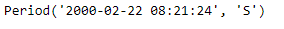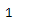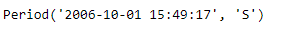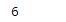Open in App
Not now

# Python | Pandas Period.weekday

• Last Updated : 06 Jan, 2019

Python is a great language for doing data analysis, primarily because of the fantastic ecosystem of data-centric python packages. Pandas is one of those packages and makes importing and analyzing data much easier.

Pandas` Period.weekday` attribute returns an integer value indicating the weekday of the given period object.

Syntax : Period.weekday

Parameters : None

Return : weekday

Example #1: Use `Period.weekday` attribute to find out the weekday in the given period object.

 `# importing pandas as pd``import` `pandas as pd`` ` `# Create the Period object``prd ``=` `pd.Period(freq ``=``'S'``, year ``=` `2000``, month ``=` `2``, day ``=` `22``,``                          ``hour ``=` `8``, minute ``=` `21``, second ``=` `24``)`` ` `# Print the Period object``print``(prd)`

Output :Now we will use the `Period.weekday` attribute to find the weekday in prd object.

 `# return the weekday``prd.weekday`

Output :As we can see in the output, the `Period.weekday `attribute has returned 1 indicating the given period is the first day of the week.

Example #2: Use `Period.weekday` attribute to find out the weekday in the given period object.

 `# importing pandas as pd``import` `pandas as pd`` ` `# Create the Period object``prd ``=` `pd.Period(freq ``=``'S'``, year ``=` `2006``, month ``=` `10``,``               ``hour ``=` `15``, minute ``=` `49``, second ``=` `17``)`` ` `# Print the object``print``(prd)`

Output :Now we will use the `Period.weekday` attribute to find the weekday in prd object.

 `# return the weekday``prd.weekday`

Output :As we can see in the output, the `Period.weekday `attribute has returned 6 indicating the given period is the sixth day of the week.

My Personal Notes arrow_drop_up